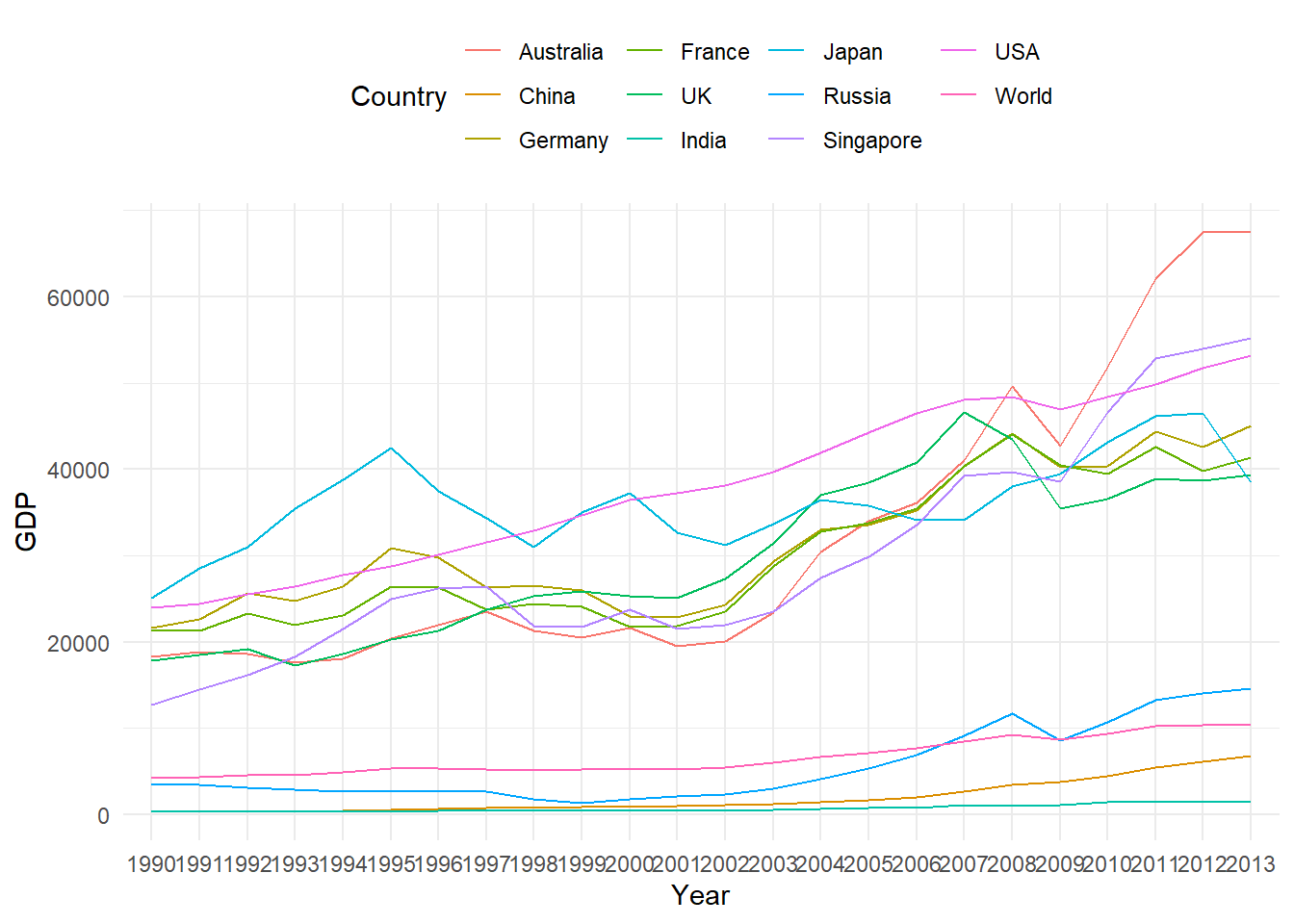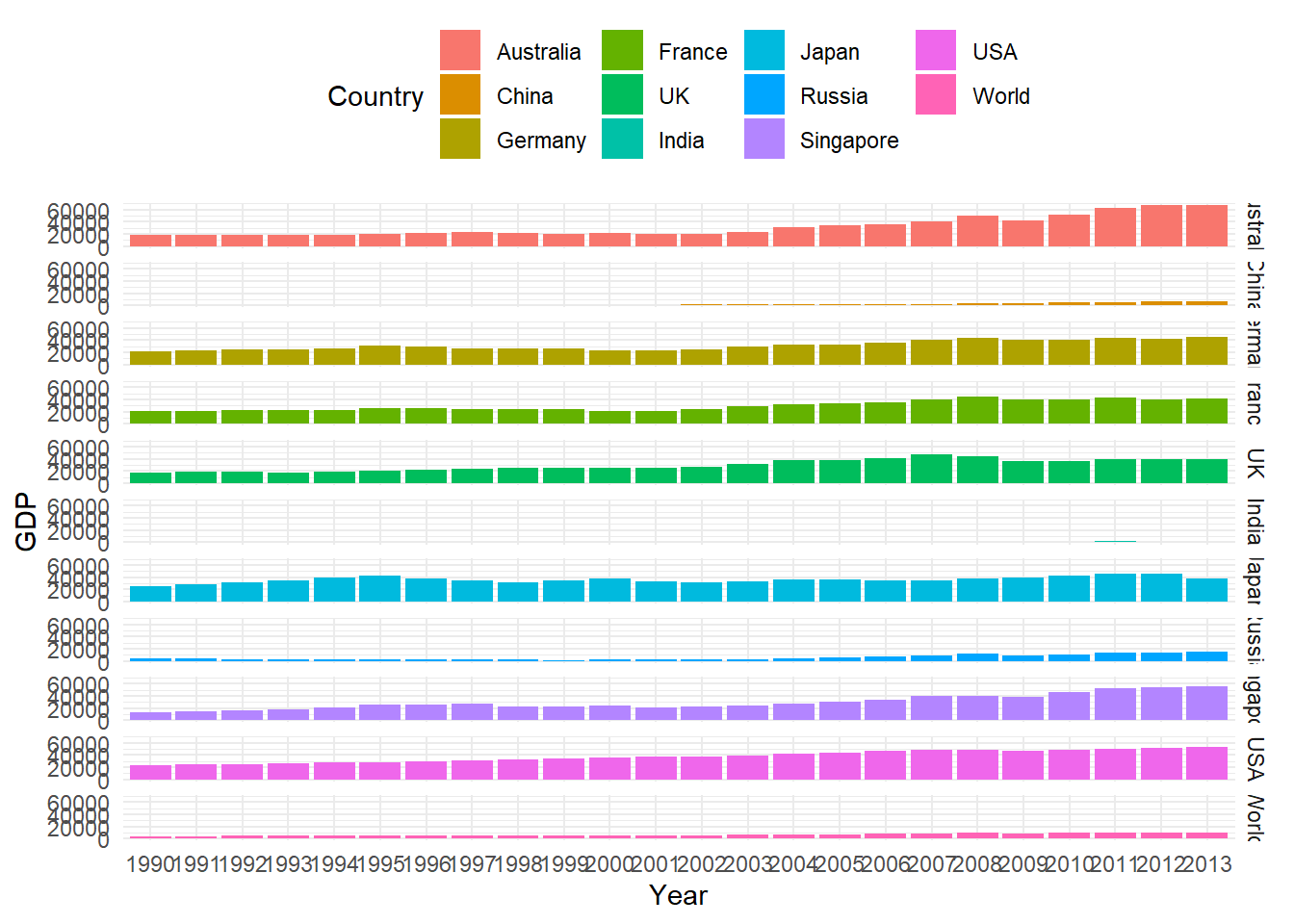# Topic 9 Panel Regression

• Panel data or longitudinal data is a data structure which contains individuals/variables (e.g., persons, firms, countries, cities etc) observed at several points in time (days, months, years, quarters etc).
• The dataset GDP_l.RData is an example of panel data where each country’s GDP is recorded over several years in time.
load("data/GDP_l.RData")
# data snapshot
GDP_l[c(1:5, 25:29, 241:245), ]
    Year   Country        GDP
1   1990 Australia 18247.3946
2   1991 Australia 18837.1893
3   1992 Australia 18599.0012
4   1993 Australia 17658.0794
5   1994 Australia 18080.6975
25  1990     China   314.4310
26  1991     China   329.7491
27  1992     China   362.8081
28  1993     China   373.8003
29  1994     China   469.2128
241 1990     World  4220.6460
242 1991     World  4357.3096
243 1992     World  4591.0928
244 1993     World  4604.2533
245 1994     World  4882.0794
• Some visualisation

• Line Chart

library(ggplot2)
p1 = ggplot(GDP_l, aes(Year, GDP, group = Country))

p1 + geom_path(aes(color = Country)) + theme_minimal() + theme(legend.position = "top")Figure 9.1: Panel Data Line Chart

• Bar Chart
p1 + geom_col(aes(fill = Country)) + theme_minimal() + theme(legend.position = "top")Figure 9.2: Panel Data Bar Chart

• Bar Chart for each country
p1 + geom_col(aes(fill = Country)) + facet_grid(Country ~ .) + theme_minimal() +
theme(legend.position = "top")Figure 9.3: Panel Data Bar Chart

• Box plot
p2 = ggplot(GDP_l, aes(Country, GDP))
p2 + geom_boxplot(aes(fill = Country)) + theme_minimal() + theme(legend.position = "top")Figure 9.4: Panel Data Box Plot

• The GDP data here has a balanced panel structure, where all the variables have values for all points in time.

• This chapter discussed the two basic panel regression models viz, Fixed Effect Model and Random Effect Model for balanced panel data.

• For an extensive discussion see econometrics textbooks including ,, and .

• The package plm provides methods for calculating these models, which will be used for in illustrative code.

• We will use the very popular Grunfeld panel dataset available in the plm package for demostration which are based on similar examples in and .

### References

Baltagi, Badi. 2005. Econometric Analysis of Panel Data. 3rd ed. John Wiley & Sons.
Croissant, Yves, and Giovanni Millo. 2008. “Panel Data Econometrics in r: The Plm Package.” Journal of Statistical Software 27 (2): 1–43.
Greene, William H. 2008. Econometric Analysis. Granite Hill Publishers.
Grunfeld, Yehuda. 1958. “The Determinants of Corporate Investment: A Study of a Number of Large Corporations in the United States.” PhD thesis, Department of Photoduplication, University of Chicago Library.
Kleiber, Christian, and Achim Zeileis. 2008. Applied Econometrics with r. Springer Science & Business Media.
Stock, James H, and Mark W Watson. 2012. Introduction to Econometrics: Global Edition. Pearson Education.
Wooldridge, Jeffrey M. 2010. Econometric Analysis of Cross Section and Panel Data. MIT press.